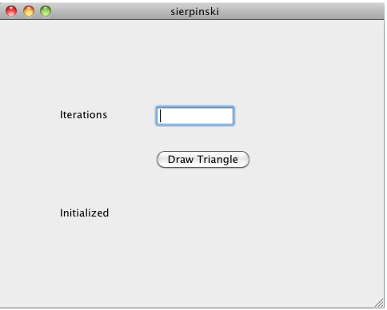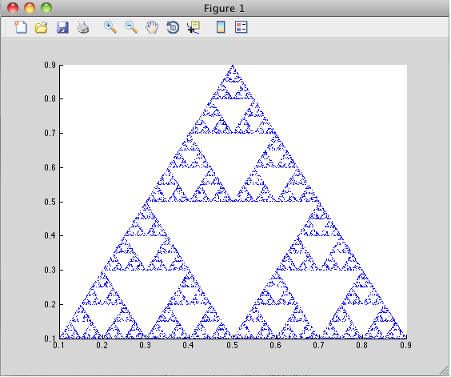## Write Applications for macOS

### Objective-C/C++ Applications for Apple’s Cocoa API

Apple Xcode, implemented in the Objective-C language, is used to develop applications using the Cocoa framework, the native object-oriented API for the Mac OS X operating system.

This article details how to deploy a graphical MATLAB® application with Objective C and Cocoa, and then deploy it using MATLAB Compiler™.

### Where’s the Example Code?

You can find example Apple Xcode, header, and project files in `matlabroot/extern/examples/compilersdk/c_cpp/triangle/code`.

### Preparing Your Apple Xcode Development Environment

To run this example, you should have prior experience with the Apple Xcode development environment and the Cocoa framework.

1. Build the shared library with MATLAB Compiler using either the Library Compiler or `mcc`.

2. Compile application code against the library’s header file and link the application against the component library and `libmwmclmcrrt`. For information about `libmwmclmcrrt` and MATLAB Runtime, see Problems Setting MATLAB Runtime Paths (MATLAB Compiler).

3. In your Apple Xcode project:

• Specify `mcc` in the project target (Build Component Library in the example code).

• Specify target settings in `HEADER_SEARCH_PATHS`.

• Specify directories containing the library header.

• Specify the path `matlabroot/extern/include`.

• Define `MWINSTALL_ROOT`, which establishes the install route using a relative path.

• Set `LIBRARY_SEARCH_PATHS` to any directories containing the shared library, as well as to the path `matlabroot/runtime/maci64`.

### Build and Run the Sierpinski Application

In this example, you deploy the graphical Sierpinski function (`sierpinski.m`, located at `matlabroot/extern/examples/compilersdk/c_cpp/triangle`).

```function [x, y] = sierpinski(iterations, draw) % SIERPINSKI Calculate (optionally draw) the points % in Sierpinski's triangle % Copyright 2004 The MathWorks, Inc. % Three points defining a nice wide triangle points = [0.5 0.9 ; 0.1 0.1 ; 0.9 0.1]; % Select an initial point current = rand(1, 2); % Create a figure window if (draw == true) f = figure; hold on; end % Pre-allocate space for the results, to improve performance x = zeros(1,iterations); y = zeros(1,iterations); % Iterate for i = 1:iterations % Select point at random index = floor(rand * 3) + 1; % Calculate midpoint between current point and random point current(1) = (current(1) + points(index, 1)) / 2; current(2) = (current(2) + points(index, 2)) / 2; % Plot that point if draw, line(current(1),current(2));, end x(i) = current(1); y(i) = current(2); end if (draw) drawnow; end ```
1. Using the Mac Finder, locate the Apple Xcode project (`matlabroot/extern/examples/compilersdk/c_cpp/triangle/xcode`). Copy files to a working directory to run this example, if needed.

2. Open `sierpinski.xcodeproj`. The development environment starts.

3. In the Groups and Files pane, select Targets.

4. Click Build and Run. The make file runs that launches MATLAB Compiler (`mcc`).

### Running the Sierpinski Application

Run the Sierpinski application from the build output directory. The following GUI appears:

MATLAB Sierpinski Function Implemented in the Mac Cocoa Environment1. In the Iterations field, enter an integer such as `10000`:2. Click Draw Triangle. The following figure appears:##### SupportGet trial now